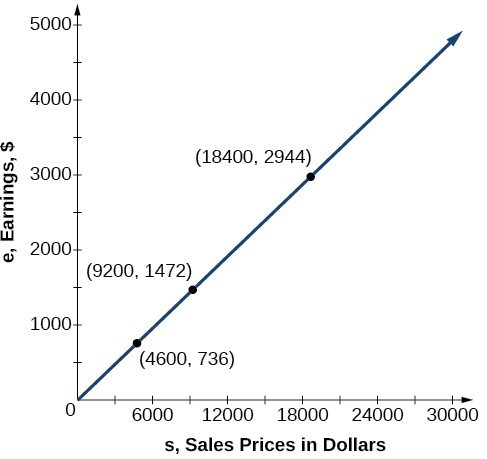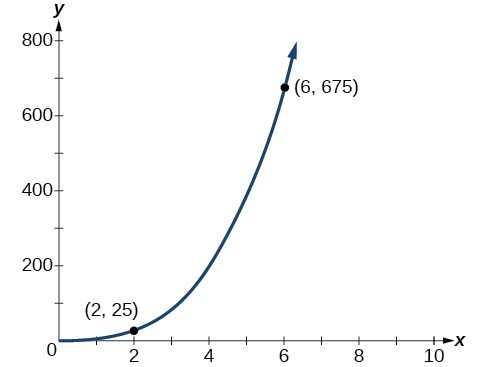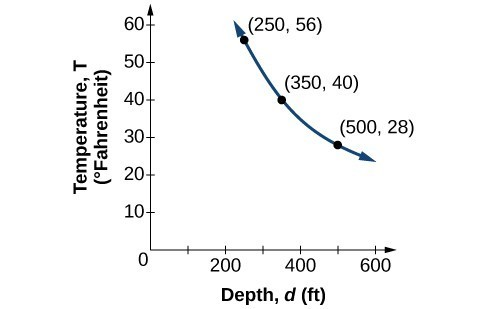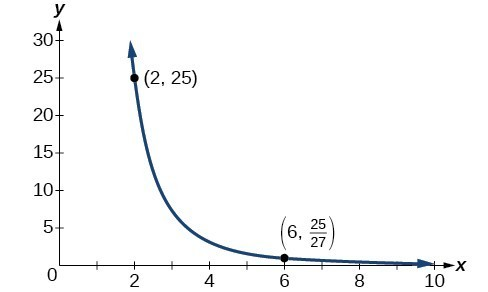Modeling Using Variation

LEARNING OBJECTIVES

By the end of this lesson, you will be able to:

• Solve direct variation problems.
• Solve inverse variation problems.
• Solve problems involving joint variation.

A used-car company has just offered their best candidate, Nicole, a position in sales. The position offers 16% commission on her sales. Her earnings depend on the amount of her sales. For instance, if she sells a vehicle for $4,600, she will earn$736. She wants to evaluate the offer, but she is not sure how. In this section, we will look at relationships, such as this one, between earnings, sales, and commission rate.

Solve direct variation problems

In the example above, Nicole’s earnings can be found by multiplying her sales by her commission. The formula e = 0.16s tells us her earnings, e, come from the product of 0.16, her commission, and the sale price of the vehicle. If we create a table, we observe that as the sales price increases, the earnings increase as well, which should be intuitive.

s, sales prices e = 0.16s Interpretation
$4,600 = 0.16(4,600) = 736 A sale of a$4,600 vehicle results in $736 earnings.$9,200 = 0.16(9,200) = 1,472 A sale of a $9,200 vehicle results in$1472 earnings.
$18,400 = 0.16(18,400) = 2,944 A sale of a$18,400 vehicle results in $2944 earnings. Notice that earnings are a multiple of sales. As sales increase, earnings increase in a predictable way. Double the sales of the vehicle from$4,600 to $9,200, and we double the earnings from$736 to \$1,472. As the input increases, the output increases as a multiple of the input. A relationship in which one quantity is a constant multiplied by another quantity is called direct variation. Each variable in this type of relationship varies directly with the other.

The graph below represents the data for Nicole’s potential earnings. We say that earnings vary directly with the sales price of the car. The formula $y=k{x}^{n}$ is used for direct variation. The value k is a nonzero constant greater than zero and is called the constant of variation. In this case, = 0.16 and = 1.Figure 1

A General Note: Direct Variation

If x and y are related by an equation of the form

$y=k{x}^{n}$

then we say that the relationship is direct variation and y varies directly with the nth power of x. In direct variation relationships, there is a nonzero constant ratio $k=\frac{y}{{x}^{n}}$, where k is called the constant of variation, which help defines the relationship between the variables.

How To: Given a description of a direct variation problem, solve for an unknown.

1. Identify the input, x, and the output, y.
2. Determine the constant of variation. You may need to divide y by the specified power of x to determine the constant of variation.
3. Use the constant of variation to write an equation for the relationship.
4. Substitute known values into the equation to find the unknown.

Example 1: Solving a Direct Variation Problem

The quantity y varies directly with the cube of x. If = 25 when = 2, find y when x is 6.

Solution

The general formula for direct variation with a cube is $y=k{x}^{3}$. The constant can be found by dividing y by the cube of x.

$\begin{cases} k=\frac{y}{{x}^{3}} \\ =\frac{25}{{2}^{3}}\\ =\frac{25}{8}\end{cases}$

Now use the constant to write an equation that represents this relationship.

$y=\frac{25}{8}{x}^{3}$

Substitute x = 6 and solve for y.

$\begin{cases}y=\frac{25}{8}{\left(6\right)}^{3}\hfill \\ \text{ }=675\hfill \end{cases}$

Analysis of the Solution

The graph of this equation is a simple cubic, as shown below.Figure 2

Q & A

Do the graphs of all direct variation equations look like Example 1?

No. Direct variation equations are power functions—they may be linear, quadratic, cubic, quartic, radical, etc. But all of the graphs pass through (0, 0).

Try It 1

The quantity y varies directly with the square of x. If = 24 when = 3, find y when x is 4.

Solution

Solve inverse variation problems

Water temperature in an ocean varies inversely to the water’s depth. Between the depths of 250 feet and 500 feet, the formula $T=\frac{14,000}{d}$ gives us the temperature in degrees Fahrenheit at a depth in feet below Earth’s surface. Consider the Atlantic Ocean, which covers 22% of Earth’s surface. At a certain location, at the depth of 500 feet, the temperature may be 28°F.

If we create a table we observe that, as the depth increases, the water temperature decreases.

d, depth $T=\frac{\text{14,000}}{d}$ Interpretation
500 ft $\frac{14,000}{500}=28$ At a depth of 500 ft, the water temperature is 28° F.
350 ft $\frac{14,000}{350}=40$ At a depth of 350 ft, the water temperature is 40° F.
250 ft $\frac{14,000}{250}=56$ At a depth of 250 ft, the water temperature is 56° F.

We notice in the relationship between these variables that, as one quantity increases, the other decreases. The two quantities are said to be inversely proportional and each term varies inversely with the other. Inversely proportional relationships are also called inverse variations.

For our example, the graph depicts the inverse variation. We say the water temperature varies inversely with the depth of the water because, as the depth increases, the temperature decreases. The formula $y=\frac{k}{x}$ for inverse variation in this case uses = 14,000.Figure 3

A General Note: Inverse Variation

If x and y are related by an equation of the form

$y=\frac{k}{{x}^{n}}$

where k is a nonzero constant, then we say that y varies inversely with the nth power of x. In inversely proportional relationships, or inverse variations, there is a constant multiple $k={x}^{n}y$.

Example 2: Writing a Formula for an Inversely Proportional Relationship

A tourist plans to drive 100 miles. Find a formula for the time the trip will take as a function of the speed the tourist drives.

Solution

Recall that multiplying speed by time gives distance. If we let t represent the drive time in hours, and v represent the velocity (speed or rate) at which the tourist drives, then vt = distance. Because the distance is fixed at 100 miles, vt = 100. Solving this relationship for the time gives us our function.

$\begin{cases}t\left(v\right)=\frac{100}{v}\hfill \\ \text{ }=100{v}^{-1}\hfill \end{cases}$

We can see that the constant of variation is 100 and, although we can write the relationship using the negative exponent, it is more common to see it written as a fraction.

How To: Given a description of an indirect variation problem, solve for an unknown.

1. Identify the input, x, and the output, y.
2. Determine the constant of variation. You may need to multiply y by the specified power of x to determine the constant of variation.
3. Use the constant of variation to write an equation for the relationship.
4. Substitute known values into the equation to find the unknown.

Example 3: Solving an Inverse Variation Problem

A quantity y varies inversely with the cube of x. If = 25 when = 2, find y when x is 6.

Solution

The general formula for inverse variation with a cube is $y=\frac{k}{{x}^{3}}$. The constant can be found by multiplying y by the cube of x.

$\begin{cases}k={x}^{3}y\hfill \\ \text{ }={2}^{3}\cdot 25\hfill \\ \text{ }=200\hfill \end{cases}$

Now we use the constant to write an equation that represents this relationship.

$\begin{cases}y=\frac{k}{{x}^{3}},k=200\hfill \\ y=\frac{200}{{x}^{3}}\hfill \end{cases}$

Substitute = 6 and solve for y.

$\begin{cases}y=\frac{200}{{6}^{3}}\hfill \\ \text{ }=\frac{25}{27}\hfill \end{cases}$

Analysis of the Solution

The graph of this equation is a rational function.Figure 4

Try It 2

A quantity y varies inversely with the square of x. If = 8 when = 3, find y when x is 4.

Solution

Solve problems involving joint variation

Many situations are more complicated than a basic direct variation or inverse variation model. One variable often depends on multiple other variables. When a variable is dependent on the product or quotient of two or more variables, this is called joint variation. For example, the cost of busing students for each school trip varies with the number of students attending and the distance from the school. The variable c, cost, varies jointly with the number of students, n, and the distance, d.

A General Note: Joint Variation

Joint variation occurs when a variable varies directly or inversely with multiple variables.

For instance, if x varies directly with both y and z, we have = kyz. If x varies directly with y and inversely with z, we have $x=\frac{ky}{z}$. Notice that we only use one constant in a joint variation equation.

Example 4: Solving Problems Involving Joint Variation

A quantity x varies directly with the square of y and inversely with the cube root of z. If = 6 when = 2 and = 8, find x when = 1 and = 27.

Solution

Begin by writing an equation to show the relationship between the variables.

$x=\frac{k{y}^{2}}{\sqrt{z}}$

Substitute = 6, = 2, and = 8 to find the value of the constant k.

$\begin{cases}6=\frac{k{2}^{2}}{\sqrt{8}}\hfill \\ 6=\frac{4k}{2}\hfill \\ 3=k\hfill \end{cases}$

Now we can substitute the value of the constant into the equation for the relationship.

$x=\frac{3{y}^{2}}{\sqrt{z}}$

To find x when = 1 and = 27, we will substitute values for y and z into our equation.

$\begin{cases}x=\frac{3{\left(1\right)}^{2}}{\sqrt{27}}\hfill \\ \text{ }=1\hfill \end{cases}$

Try It 3

x varies directly with the square of y and inversely with z. If = 40 when = 4 and = 2, find x when = 10 and = 25.

Solution

Key Equations

 Direct variation $y=k{x}^{n},k\text{ is a nonzero constant}$. Inverse variation $y=\frac{k}{{x}^{n}},k\text{ is a nonzero constant}$.

Key Concepts

• A relationship where one quantity is a constant multiplied by another quantity is called direct variation.
• Two variables that are directly proportional to one another will have a constant ratio.
• A relationship where one quantity is a constant divided by another quantity is called inverse variation.
• Two variables that are inversely proportional to one another will have a constant multiple.
• In many problems, a variable varies directly or inversely with multiple variables. We call this type of relationship joint variation.

Glossary

constant of variation
the non-zero value k that helps define the relationship between variables in direct or inverse variation
direct variation
the relationship between two variables that are a constant multiple of each other; as one quantity increases, so does the other
inverse variation
the relationship between two variables in which the product of the variables is a constant
inversely proportional
a relationship where one quantity is a constant divided by the other quantity; as one quantity increases, the other decreases
joint variation
a relationship where a variable varies directly or inversely with multiple variables
varies directly
a relationship where one quantity is a constant multiplied by the other quantity
varies inversely
a relationship where one quantity is a constant divided by the other quantity

Section Exercises

1. What is true of the appearance of graphs that reflect a direct variation between two variables?

2. If two variables vary inversely, what will an equation representing their relationship look like?

3. Is there a limit to the number of variables that can jointly vary? Explain.

For the following exercises, write an equation describing the relationship of the given variables.

4. y varies directly as x and when = 6, = 12.

5. y varies directly as the square of x and when x = 4, y = 80

6. y varies directly as the square root of x and when = 36, = 24.

7. y varies directly as the cube of x and when = 36, = 24.

8. y varies directly as the cube root of x and when = 27, = 15.

9. y varies directly as the fourth power of x and when = 1, = 6.

10. y varies inversely as x and when = 4, = 2.

11. y varies inversely as the square of x and when = 3, = 2.

12. y varies inversely as the cube of x and when = 2, = 5.

13. y varies inversely as the fourth power of x and when = 3, = 1.

14. y varies inversely as the square root of x and when = 25, = 3.

15. y varies inversely as the cube root of x and when = 64, = 5.

16. y varies jointly with x and z and when = 2 and = 3, = 36.

17. y varies jointly as x, z, and w and when = 1, = 2, = 5, then = 100.

18. y varies jointly as the square of x and the square of z and when = 3 and = 4, then y = 72.

19. y varies jointly as x and the square root of z and when = 2 and = 25, then = 100.

20. y varies jointly as the square of x the cube of z and the square root of w. When = 1, = 2, and = 36, then = 48.

21. y varies jointly as x and z and inversely as w. When = 3, = 5, and = 6, then = 10.

22. y varies jointly as the square of x and the square root of z and inversely as the cube of w. When = 3, = 4, and = 3, then = 6.

23. y varies jointly as x and z and inversely as the square root of w and the square of t. When = 3, = 1, = 25, and = 2, then = 6.

For the following exercises, use the given information to find the unknown value.

24. y varies directly as x. When = 3, then = 12. Find y when = 20.

25. y varies directly as the square of x. When = 2, then = 16. Find y when = 8.

26. y varies directly as the cube of x. When = 3, then = 5. Find y when = 4.

27. y varies directly as the square root of x. When = 16, then = 4. Find y when = 36.

28. y varies directly as the cube root of x. When = 125, then = 15. Find y when = 1,000.

29. y varies inversely with x. When = 3, then = 2. Find y when = 1.

30. y varies inversely with the square of x. When = 4, then = 3. Find y when = 2.

31. y varies inversely with the cube of x. When = 3, then = 1. Find y when = 1.

32. y varies inversely with the square root of x. When = 64, then = 12. Find y when = 36.

33. y varies inversely with the cube root of x. When = 27, then = 5. Find y when = 125.

34. y varies jointly as x and z. When = 4 and = 2, then = 16. Find y when = 3 and = 3.

35. y varies jointly as x, z, and w. When = 2, = 1, and = 12, then = 72. Find y when = 1, = 2, and = 3.

36. y varies jointly as x and the square of z. When = 2 and = 4, then = 144. Find y when = 4 and = 5.

37. y varies jointly as the square of x and the square root of z. When = 2 and = 9, then = 24. Find y when = 3 and = 25.

38. y varies jointly as x and z and inversely as w. When = 5, = 2, and = 20, then = 4. Find y when = 3 and = 8, and = 48.

39. y varies jointly as the square of x and the cube of z and inversely as the square root of w. When = 2, = 2, and = 64, then = 12. Find y when = 1, = 3, and = 4.

40. y varies jointly as the square of x and of z and inversely as the square root of w and of t. When = 2, = 3, = 16, and = 3, then = 1. Find y when = 3, = 2, = 36, and = 5.

For the following exercises, use a calculator to graph the equation implied by the given variation.

41. y varies directly with the square of x and when = 2, = 3.

42. y varies directly as the cube of x and when = 2, = 4.

43. y varies directly as the square root of x and when = 36, = 2.

44. y varies inversely with x and when = 6, = 2.

45. y varies inversely as the square of x and when = 1, = 4.

For the following exercises, use Kepler’s Law, which states that the square of the time, T, required for a planet to orbit the Sun varies directly with the cube of the mean distance, a, that the planet is from the Sun.

46. Using the Earth’s time of 1 year and mean distance of 93 million miles, find the equation relating and a.

47. Use the result from the previous exercise to determine the time required for Mars to orbit the Sun if its mean distance is 142 million miles.

48. Using Earth’s distance of 150 million kilometers, find the equation relating T and a.

49. Use the result from the previous exercise to determine the time required for Venus to orbit the Sun if its mean distance is 108 million kilometers.

50. Using Earth’s distance of 1 astronomical unit (A.U.), determine the time for Saturn to orbit the Sun if its mean distance is 9.54 A.U.

For the following exercises, use the given information to answer the questions.

51. The distance s that an object falls varies directly with the square of the time, t, of the fall. If an object falls 16 feet in one second, how long for it to fall 144 feet?

52. The velocity v of a falling object varies directly to the time, t, of the fall. If after 2 seconds, the velocity of the object is 64 feet per second, what is the velocity after 5 seconds?

53. The rate of vibration of a string under constant tension varies inversely with the length of the string. If a string is 24 inches long and vibrates 128 times per second, what is the length of a string that vibrates 64 times per second?

54. The volume of a gas held at constant temperature varies indirectly as the pressure of the gas. If the volume of a gas is 1200 cubic centimeters when the pressure is 200 millimeters of mercury, what is the volume when the pressure is 300 millimeters of mercury?

55. The weight of an object above the surface of the Earth varies inversely with the square of the distance from the center of the Earth. If a body weighs 50 pounds when it is 3960 miles from Earth’s center, what would it weigh it were 3970 miles from Earth’s center?

56. The intensity of light measured in foot-candles varies inversely with the square of the distance from the light source. Suppose the intensity of a light bulb is 0.08 foot-candles at a distance of 3 meters. Find the intensity level at 8 meters.

57. The current in a circuit varies inversely with its resistance measured in ohms. When the current in a circuit is 40 amperes, the resistance is 10 ohms. Find the current if the resistance is 12 ohms.

58. The force exerted by the wind on a plane surface varies jointly with the square of the velocity of the wind and with the area of the plane surface. If the area of the surface is 40 square feet surface and the wind velocity is 20 miles per hour, the resulting force is 15 pounds. Find the force on a surface of 65 square feet with a velocity of 30 miles per hour.

59. The horsepower (hp) that a shaft can safely transmit varies jointly with its speed (in revolutions per minute (rpm) and the cube of the diameter. If the shaft of a certain material 3 inches in diameter can transmit 45 hp at 100 rpm, what must the diameter be in order to transmit 60 hp at 150 rpm?

60. The kinetic energy K of a moving object varies jointly with its mass and the square of its velocity v. If an object weighing 40 kilograms with a velocity of 15 meters per second has a kinetic energy of 1000 joules, find the kinetic energy if the velocity is increased to 20 meters per second.